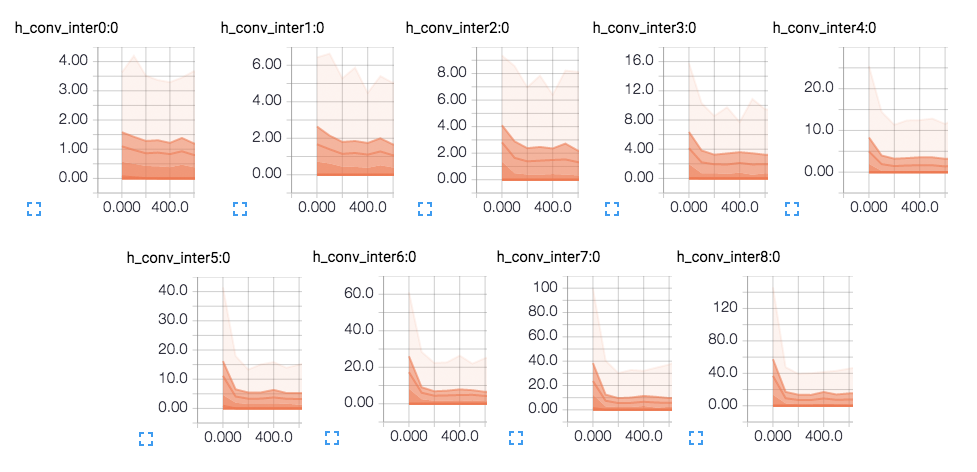# Proper Initialization of Weights for DCNNs

Originally posted 2016-09-02

Lately, I’ve been working on a neural network, based on AlphaGo’s policy network. To test it, I’ve been using smaller parameters (width of each layer, as well as number of layers), for faster execution times. All was fine, and I was getting decent accuracy results, even with the smaller test networks. But when it came time to scale the network up to the full size, I started running into all sorts of NaN errors. I experimented with scaling up in increments until I started running into NaN errors; they seemed to be triggered both by increasing the number of neurons in a layer, as well as number of layers. I was stuck for a long time, until I happened to notice my activations in TensorBoard.Each graph shows the 50/69/84/93/100th percentiles of the neuron activations for each layer. (The numbers correspond to 0, 0.5, 1, 1.5 standard deviations from mean. The 50th percentile is approximately 0, because ReLU neurons will discard negative values.) I realized that the average magnitude of the activations is growing exponentially with each layer, with a growth factor of about 1.5.

Why is this happening?

In my neural network, each layer contains 19x19xK neurons, where K was 64 for the above graphs. Because I’m using a convolutional NN, each neuron receives a 3x3xK slice from the previous layer as its inputs - so in this case, 576 inputs. If the inputs have a variance of 1 (stddev of sqrt(1) = 1), then their weighted sum has variance 576 (stddev of sqrt(576) = 24). That being said, the weights in the weighted sum are not all 1 - they were randomly initialized by selecting from a Gaussian distribution with hardcoded stddev 0.1. So, all in all, each layer ends up scaling up by a factor of about 2.4. That is approximately the scaling ratio observed; the discrepancy can be attributed to ReLU neurons randomly setting half of the outputs as 0.

Not shown in the graphs is the backpropagation step, which for ReLU neurons, continues to incur a scaling factor of 2.4 for each backwards layer. Thus, you can expect your very first layer to move by sqrt(NUM_INPUTS_PER_NEURON)^(2 * NUM_LAYERS) during each step of training!

Thus, as I scaled wider and deeper, this exponential law ended up blowing up and generating infinities (NaN).

The fix was to figure out how many inputs each neuron had, and draw the weights for that neuron from a Gaussian having standard deviation equal to 1 / sqrt(9 * K) - K being the width of the layer. That fixes the base of the exponent as approximately 1, and the exponential never goes anywhere.